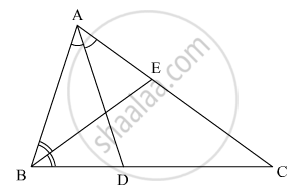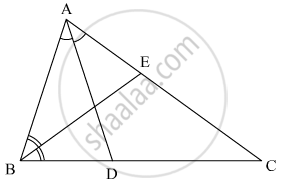Advertisement Remove all ads

# In the given figure, ABC is a triangle in which ∠B = 2∠C. D is a point on side BC such that AD bisects ∠BAC and AB = CD. BE is the bisector of ∠B. The measure of ∠BAC is - Mathematics

MCQ

In the given figure, ABC is a triangle in which ∠B = 2∠C. D is a point on side BC such that ADbisects ∠BAC and AB = CD. BE is the bisector of ∠B. The measure of ∠BAC is#### Options

• 72°

• 73°

• 74°

• 95°

Advertisement Remove all ads

#### Solution

It is given that

∠B = 2∠C,

AB = CD

∠BAD = DAC

∠ABE = ∠EBC

We have to find ∠BACNow AB = CD

AB = BD

Now the triangle is isosceles

∠B = 2∠C

Let

∠B = x

∠B = 2x

∠C = x

So ∠B = ∠A

Now

∠A + ∠B + ∠C = 180°

2x + 2x + x = 180°

5x = 180°

x = 36°

Since

∠A  = 2x

= `2 xx 36°

= 72°

Is there an error in this question or solution?
Advertisement Remove all ads

#### APPEARS IN

RD Sharma Mathematics for Class 9
Chapter 12 Congruent Triangles
Q 20 | Page 88
Advertisement Remove all ads
Advertisement Remove all ads
Share
Notifications

View all notifications

Forgot password?
Course